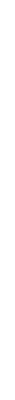1. (18 pts) Referring to the table "Relationship between Z2/r Ratios and Acidity of Metal Ions", compute the smallest and largest radius that will allow a +1 ion to fit into each category of cation acidity. Do the same for a +2, +3 and +4 cation. The referring to Table C (and a table of electronegativities) , list all ions from table C that fit into each of the categories. Locate the ions of each category on a periodic table to see what sort of periodic pattern results. 2. (12 pts) Consider the cations U3+, Ag+, As3+, Mg+2 (a) Classify the acidity of each of these cations and describe the reactions of their chlorides with water. (b) Which of these would give cloudiness or precipitation upon dissolving in water? What would you do to rectify this if it occurred? (c) Using the equations for calculating pKa in the reading notes, calculate pKa for each of the cations and determine whether any of the ions needs to be shifted to another category of acidity.